Disclosure: This post may contain affiliate links, meaning when you click the links and make a purchase, we receive a commission.

# How to Use Compound Interest Formula in Excel

Compound interest is the interest that’s calculated both on the initial principal of a deposit or loan and on all previously accumulated interest.In this article, we are going to learn the compound interest formula in Excel.

Professor Albert Einstein once said, “Compounding interest is the most powerful force in the universe”. It is doubtful that Einstein really said that or not, but it doesn’t take away from the importance of the message. Compounding is a very powerful force that either work against you (borrowing) or for you (investing).

Let’s get started with the basics of Compound Interest.

## Knowing the Compound Interest Formula

For calculating the future value of any investment earning at a constant rate of interest the following formula can be used. Which is,

Future Value = P*(1+r)^n

Where,

• P-initial amount invested
• r -annual interest rate (as a decimal or a percentage)
• n-number of periods over which the investment is made

For example, if in 5 years you invest \$100 at a rate of 5%. Then the calculated future value is, 100*(1+5%)^5 = 127.6282

## Compound Interest Formula in Excel

Here we are going to calculate the future value of some venture using the formula of compound interest in excel. Let`s say we have a table that states \$100 investment for 5 years at an annual interest rate of 5%. For this, we need to calculate the future value using the formula of compound interest. We can use the formula directly to calculate the future value in excel. The below picture shows how it is done.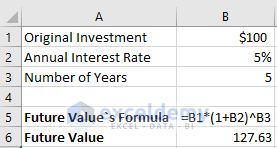As we can see the Future value is \$127.63 which is the accurate value for this.

## Calculating Compound Interest Over Multiple Years

The future value of some amount of investment for a number of years can be shown using the same formula. Here the investment goes as the years added.

The following picture shows the future value of an original investment of \$100 for different years, invested at an annual interest rate of 5%.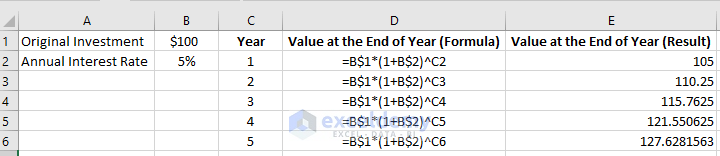## Compound Interest Formula with Monthly Contributions in Excel

If the interest is paid monthly then the formula for future value becomes, Future Value = P*(1+r/12)^(n*12).

The following picture shows the formula of compound interest to calculate the future value of any investment with monthly contributions.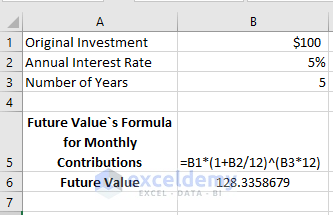## Calculating Compound Interest while Interest is Paid Quarterly

Just like the previous example If the interest on investment is paid quarterly then the formula for future value becomes, Future Value = P*(1+r/4)^(n*4).

The following picture shows, how the compound interest is calculated when the interest is paid quarterly.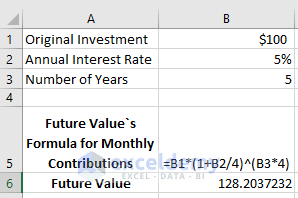## Compound Interest Formula with daily Contributions in Excel

Just like the previous examples, we can calculate the future value of the initial investment for daily contributions. Here the formula will be, Future Value = P*(1+r/365)^(n*365).

The following picture shows how it is calculated.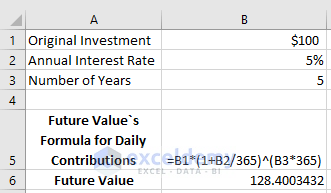As you can see, while calculating the future value for the daily compounding interest with the same investment, the result is a bit higher than with monthly compounding or yearly compounding. This is because the 5% interest rate adds interest to the initial investment each day. For monthly or yearly compounding it adds for each month and year respectively.

## Excel FV Function for Calculating Compound Interest

It’s a good practice to use Excel’s FV function which calculates the future value based on different factors. The syntax for this FV function is,

FV(rate, nper, pmt, [pv], [type])

Where,

• rate-the interest rate
• nper-number of periods for the investment
• pmt-the periodic payment
• pv-the present value/initial investment
• type is optional.

the screenshot below shows the future value of \$5,000 investment after 12 years at an annual interest rate of 6%.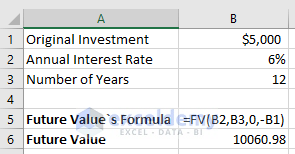Note: Here 0 is used as there is no periodic payment. We need to multiply the present value (pv) with -1 as excel treats this as “money out” for your investment.

For calculating the future value to be compounded with monthly, quarterly, weekly or daily contribution you need to divide the annual interest rate (for FV function it is rate) with the contribution and multiply the contribution with the number of years (for FV function it is nper).

Let`s say we want to calculate the future value of same factors. The only difference will be with the compounded period. Here, the compounded periods will be the total number of weeks per year which is 52. The picture below shows how it is done.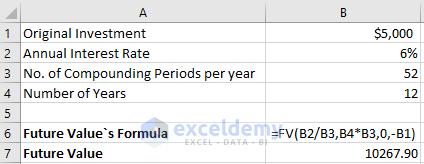Let’s calculate the compound interest with more options where additional contributions (pmet & type) are added.

We will calculate for the same example where an additional contribution of \$500 is added. The following picture shows us the whole calculation and result.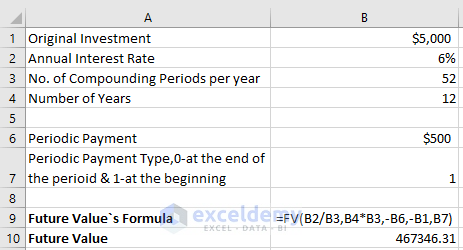Here,

• B1 – original investment
• B2 – annual interest rate
• B3 – compounding periods per year
• B4 – the number of years
• B6 – periodic payments
• B7 – periodic payments type. Put 1 if the additional amount is deposited at the beginning, put 0 if the additional amount is deposited at the end.

Note: We multiplied the periodic payment by -1. It is because like the present value (pv), excel also treats this as “money out” for your investment.

## Online Compound Interest Calculator

If you want to get the help of different online site for calculating the compound interest rather than finding it out through excel, you can try it by using the help of below websites which can help you find the compound interest the way you want.

### Compound Interest Calculator by The Calculator Site

This site may help you find different solutions of statistical analysis. It provides an online calculator for finding the compound interest on savings. It also provides you the option of compounding interval. You can include the option of regular monthly withdrawals or deposits. There is another calculator attach to it which neglects the regular deposits/withdrawals. So, you have the freedom to choose the option you want. Click on the link below to get the help of The Calculator Site.

The Calculator Site

After your calculation is done it will not only show you the result but it will also show you the graph of your result. So, you can have a clear idea about what is actually happening with the interest and balance.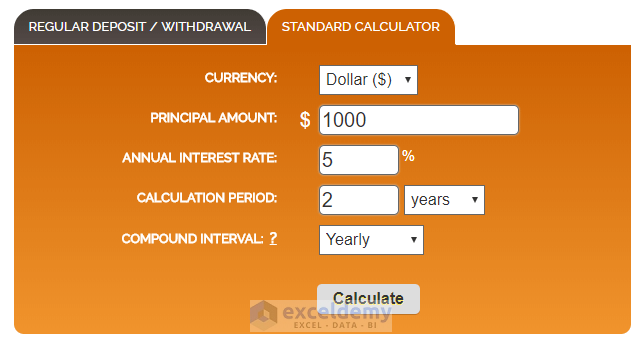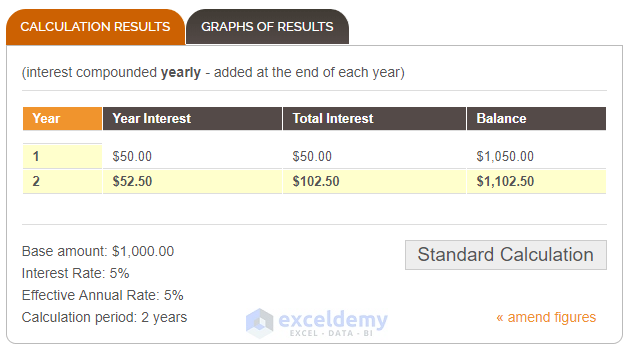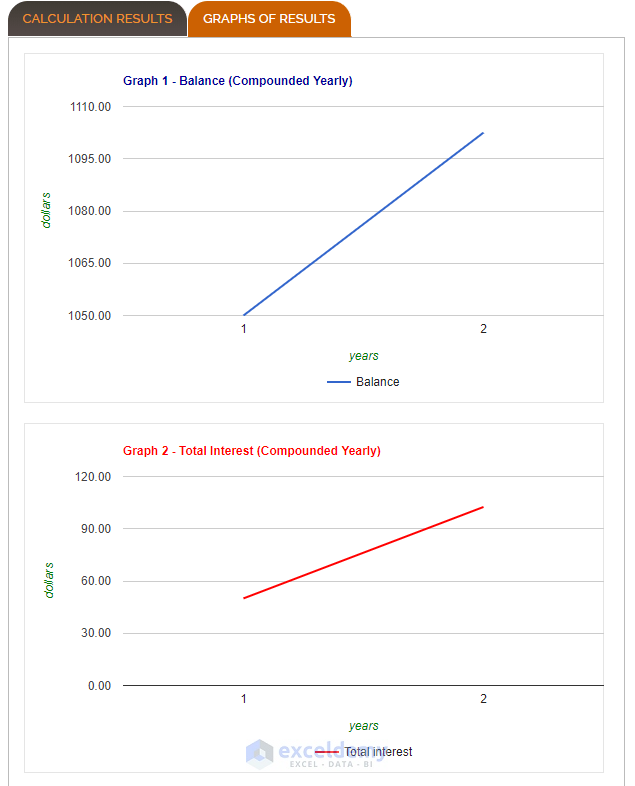### Compound Interest Calculator by Money-Zine

The online calculator from Money-Zine is probably the simplest online calculator for calculating the compound interest. It will ask for only three inputs which are, Investment or Savings Amount (\$), Investment Duration (Years) and Interest Rate Before Compounding (%). The calculator calculates different interest rate of annual, semi-annual, quarterly, monthly, weekly and daily along with the future value of the investment which was provided. Click the following link to get the help of Money-Zine.

Money-Zine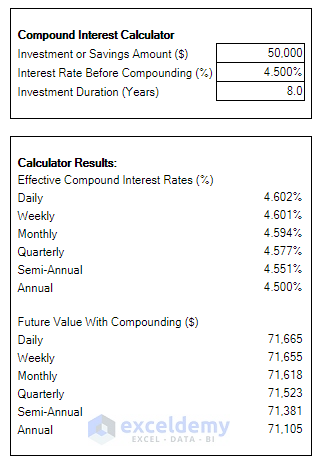### Compound Interest Calculator by Calculate Stuff

This online site calculates the compound interest briefly by taking the regular investment into consideration. You can also choose the regular investment by monthly, quarterly and annually. The compounding option can also be chosen with a unique feature of starting date.

By pressing the calculate option after inserting the values, you will see a pie-chart which indicates your initial and regular investment along with the interest for the whole years of investment. You will also see the investment growth chart for different years. For your own benefits, this site will also show you the yearly and monthly breakdown of your investments. To get the help of this website, click on the link below.

Calculate Stuff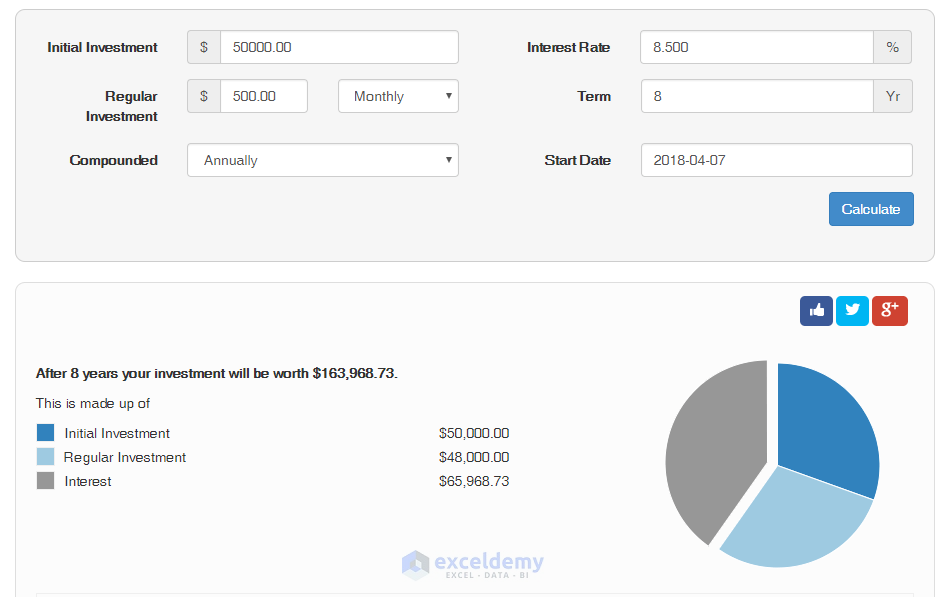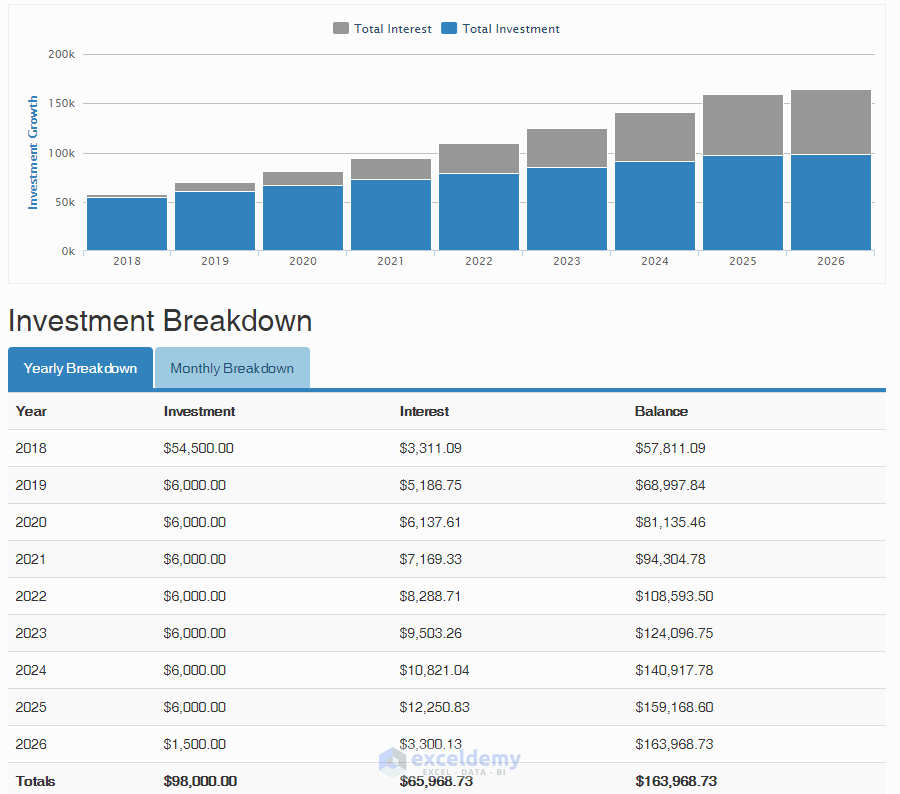## Conclusion

In this article, we mainly discuss the general formula for compound interest and how the future value of some investment and interest is calculated using the formula of compound interest. We also discuss the FV function that can be used as a compound interest calculator in excel.

So, basically you can apply the direct formula of compound interest or you can use the FV function for calculating the future value of some amount of investment based on different factors.

The Excel file in which the whole calculations are done is attached below so that you can have a brief idea about the topic.

Compound Interest Formula